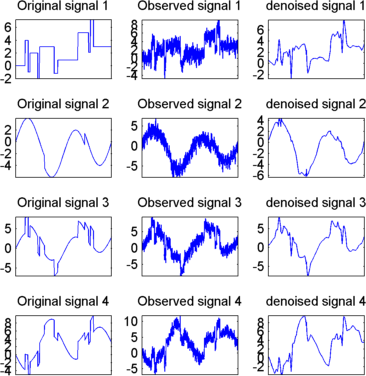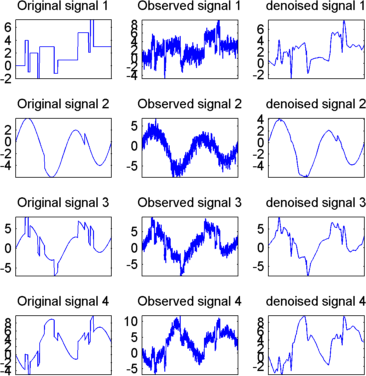## Multivariate Wavelet Denoising

This section demonstrates the features of multivariate denoising provided in the Wavelet Toolbox™ software. The toolbox includes the `wmulden` function and a Wavelet Analyzer app . This section also describes the command-line and app methods and includes information about transferring signal and parameter information between the disk and the app.

This multivariate wavelet denoising problem deals with models of the form X(t) = F(t) + e(t), where the observation X is p-dimensional, F is the deterministic signal to be recovered, and e is a spatially correlated noise signal. This kind of model is well suited for situations for which such additive, spatially correlated noise is realistic.

### Multivariate Wavelet Denoising — Command Line

This example uses noisy test signals. In this section, you will

• Display the original and observed signals.

• Remove noise by a simple multivariate thresholding after a change of basis.

• Display the original and denoised signals.

• Improve the obtained result by retaining less principal components.

• Display the number of retained principal components.

• Display the estimated noise covariance matrix.

1. Load a multivariate signal by typing the following at the MATLAB® prompt:

```load ex4mwden whos ```
NameSizeBytesClass
`covar``4x4``128``double array`
`x``1024x4``32768``double array`
`x_orig``1024x4``32768``double array`

Usually, only the matrix of data `x` is available. Here, we also have the true noise covariance matrix (`covar`) and the original signals (`x_orig`). These signals are noisy versions of simple combinations of the two original signals. The first one is “Blocks” which is irregular, and the second is “HeavySine,” which is regular except around time 750. The other two signals are the sum and the difference of the two original signals. Multivariate Gaussian white noise exhibiting strong spatial correlation is added to the resulting four signals, which leads to the observed data stored in `x`.

2. Display the original and observed signals by typing

```kp = 0; for i = 1:4 subplot(4,2,kp+1), plot(x_orig(:,i)); axis tight; title(['Original signal ',num2str(i)]) subplot(4,2,kp+2), plot(x(:,i)); axis tight; title(['Observed signal ',num2str(i)]) kp = kp + 2; end ```The true noise covariance matrix is given by

```covar covar = 1.0000 0.8000 0.6000 0.7000 0.8000 1.0000 0.5000 0.6000 0.6000 0.5000 1.0000 0.7000 0.7000 0.6000 0.7000 1.0000 ```
3. Remove noise by simple multivariate thresholding.

The denoising strategy combines univariate wavelet denoising in the basis where the estimated noise covariance matrix is diagonal with noncentered Principal Component Analysis (PCA) on approximations in the wavelet domain or with final PCA.

First, perform univariate denoising by typing the following to set the denoising parameters:

```level = 5; wname = 'sym4'; tptr = 'sqtwolog'; sorh = 's'; ```

Then, set the PCA parameters by retaining all the principal components:

```npc_app = 4; npc_fin = 4; ```

Finally, perform multivariate denoising by typing

```x_den = wmulden(x, level, wname, npc_app, npc_fin, tptr, sorh); ```
4. Display the original and denoised signals by typing

```kp = 0; for i = 1:4 subplot(4,3,kp+1), plot(x_orig(:,i)); set(gca,'xtick',[]); axis tight; title(['Original signal ',num2str(i)]) subplot(4,3,kp+2), plot(x(:,i)); set(gca,'xtick',[]); axis tight; title(['Observed signal ',num2str(i)]) subplot(4,3,kp+3), plot(x_den(:,i)); set(gca,'xtick',[]); axis tight; title(['denoised signal ',num2str(i)]) kp = kp + 3; end ```5. Improve the first result by retaining fewer principal components.

The results are satisfactory. Focusing on the two first signals, note that they are correctly recovered, but the result can be improved by taking advantage of the relationships between the signals, leading to an additional denoising effect.

To automatically select the numbers of retained principal components by Kaiser's rule (which keeps the components associated with eigenvalues exceeding the mean of all eigenvalues), type

```npc_app = 'kais'; npc_fin = 'kais'; ```

Perform multivariate denoising again by typing

```[x_den, npc, nestco] = wmulden(x, level, wname, npc_app, ... npc_fin, tptr, sorh); ```
6. Display the number of retained principal components.

The second output argument gives the numbers of retained principal components for PCA for approximations and for final PCA.

```npc npc = 2 2 ```

As expected, since the signals are combinations of two initial ones, Kaiser's rule automatically detects that only two principal components are of interest.

7. Display the estimated noise covariance matrix.

The third output argument contains the estimated noise covariance matrix:

```nestco nestco = 1.0784 0.8333 0.6878 0.8141 0.8333 1.0025 0.5275 0.6814 0.6878 0.5275 1.0501 0.7734 0.8141 0.6814 0.7734 1.0967 ```

As you can see by comparing with the true matrix covar given previously, the estimation is satisfactory.

8. Display the original and final denoised signals by typing

```kp = 0; for i = 1:4 subplot(4,3,kp+1), plot(x_orig(:,i)); set(gca,'xtick',[]); axis tight; title(['Original signal ',num2str(i)]); set(gca,'xtick',[]); axis tight; subplot(4,3,kp+2), plot(x(:,i)); set(gca,'xtick',[]); axis tight; title(['Observed signal ',num2str(i)]) subplot(4,3,kp+3), plot(x_den(:,i)); set(gca,'xtick',[]); axis tight; title(['denoised signal ',num2str(i)]) kp = kp + 3; end ```The results are better than those previously obtained. The first signal, which is irregular, is still correctly recovered, while the second signal, which is more regular, is denoised better after this second stage of PCA.